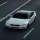Home
IT Knowledge
Inspiration
Languages
EN

# Java 8 - find first element in Stream that matches criteria

0 points
Created by:Brian-Tompset
431

In this article, we would like to show you how to find the first element in Stream that matches the criteria in Java 8.

In the example below, we use:

• `stream()` - to create a stream from the List,
• `filter()` - to specify the criteria (in our case if the element starts with "`B`"),
• `findFirst()` - to return the first value that matches the criteria,
• `orElse()` - to return null if none of the elements matches.

Practical example:

``````import java.util.*;

public class Example {

public static void main(String[] args) {
// create list of strings
List<String> letters = Arrays.asList("A1", "B1", "A2", "B2");

// find first element that matches the criteria
String result = letters.stream()
.filter(x -> x.startsWith("B")) // <----- example criteria
.findFirst()
.orElse(null);

System.out.println(result);  // B1
}
}``````

Output:

``B1``